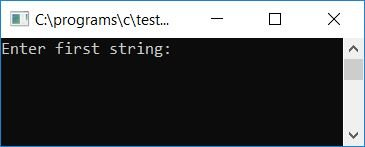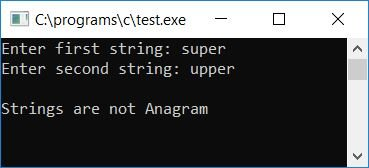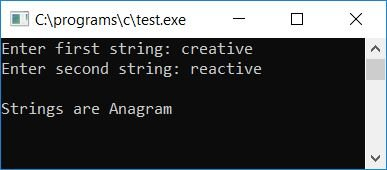# C program to check if strings are anagrams or not

In this tutorial, we will learn about how to create a program in C that checks whether any given two strings are anagrams or not.

## Check the anagram or not in C.

To check whether any given two strings (by the user at run-time) are anagrams or not in C programming, you have to ask the user to enter the two strings to check and find out whether both strings are anagrams or not, as shown in the program given below.

Two strings will be anagrams of each other if and only if they contain the same number of characters; the order of the characters doesn't matter. That is, if two strings are anagrams of each other, then one string can be rearranged to form the other string. For Example:

• abc and cba are anagrams.
• creative and reactive are anagrams.
• course and source are anagrams.

The two strings saying "super and "upper" are not anagrams. Both strings contain the same number of characters but don't contain each and every character of "super" in "upper" (or "upper" in "super," that is, you cannot rearrange the string "super" to form "upper" or "super," respectively).

Let's take a look at the program to check whether the given two strings are anagrams or not in C. Here is the C anagram program source code:

```#include<stdio.h>
#include<conio.h>
#include<string.h>
int main()
{
char str1, str2;
int len, len1, len2, i, j, found=0, not_found=0;
printf("Enter first string: ");
gets(str1);
printf("Enter second string: ");
gets(str2);
len1 = strlen(str1);
len2 = strlen(str2);
if(len1 == len2)
{
len = len1;
for(i=0; i<len; i++)
{
found = 0;
for(j=0; j<len; j++)
{
if(str1[i] == str2[j])
{
found = 1;
break;
}
}
if(found == 0)
{
not_found = 1;
break;
}
}
if(not_found == 1)
printf("\nStrings are not Anagram");
else
printf("\nStrings are Anagram");
}
else
printf("\nBoth string must contain same number of character to be an Anagram Strings");
getch();
return 0;
}```

As the above program was written in the Code::Blocks IDE, therefore, after a successful build and run, you will get the following output: Here is the initial snapshot of the sample run:Now supply any two strings, say social and soliat, as input and press the ENTER key to see the output as shown in the second snapshot of the sample run given here:Let's take another sample run and check for another two strings, say super and upper. Here is the final snapshot of the sample run:As you can see from the above output, where the two strings say super and upper, supply The first string, "super," has an extra s and one missing p. And the second string, upper, has a second p as an extra character, and one character, s, is missing from it. Therefore, we cannot rearrange super to form upper (or upper to form super).

Let's check for another two strings, say creative and reactive, using the following sample run. Here is the final snapshot of the sample run:Here are some of the main steps used in the above program:

• Receive any two strings as input.
• Use the strlen() function to find the length of both strings.
• Then initialize the length of the first string to any variable, say len1, and the length of the second string to any variable, say len2.
• Now check whether the length of both strings is equal or not.
• If both strings don't have the same number of characters, then print any message like Both strings must contain the same number of characters to be anagram strings.
• And if both strings contain the same number of characters, then follow the steps given below to check whether each and every character of the first string is present inside the second string or not.
• Create a for loop that runs from 0 to one less than the string's length (either the first or second because we have checked and found that both strings contain the same number of characters).
• Inside the for loop, initialize 0 to any variable, say found, that increments its value by 1 if a character from the first string gets found in the second string.
• Create another for loop that also runs from 0 to one less than the string length.
• Here we have created two for loops in a way to compare each and every character of the first string to each and every character of the second string.
• While comparing, if a character from the first string gets matched to the character of the second string, then initialize 1 to the found variable and break out of the inner for loop using the break keyword.
• After running the inner for loop, check whether found holds its original value of 0 or not.
• If it holds, then the current character of the first string is not found in the second string. Therefore, we have checked after each and every complete run of the inner for loop to see whether the value found holds its original value or not. If it holds (that is, 0), then we have to initialize 1 to the not_found variable. And get out of the outer for loop. As if any of the characters from the first string does not get found in the second, it means it is not required to check for the remaining characters, as the strings cannot be anagrams of each other if any character from the first string does not get matched with a character in the second string.
• At last, we have checked to see whether the variable say not_found holds its original value of 0 or not.
• If it holds, then both strings are anagrams. Otherwise, both strings are not anagrams (in case it holds the value of 1).

The brief description of the above program is that first we have to check the length of both strings; if length is equal, then proceed further; otherwise, print the message for unequal length. We have compared the first character of the first string to all the characters of the second string one by one, then compared the second character of the first string to all the characters of the second string one by one, then compared the third character of the first string to all the characters of the second string one by one, and so on.

While comparing, if any of the characters of the first string match the characters of the second string, then we have initialized 1 to the found and exit from the inner loop. If there is no match found for any character, then 1 will not be initialized to it. So the found holds its previous or initial value, which is 0.

And after exiting the inner loop, on checking "if (found == 0)", it becomes true, and 1 will be initialized to the variable not_found and exit from the outer loop. Now, after exiting the outer loop, we have to check whether not_found will be equal to 1 or 0. If not_found is equal to 1, then both strings are not anagrams, and if not_found is equal to 0, then both strings will be anagrams.

#### The same program in different languages

C Quiz

« Previous Program Next Program »